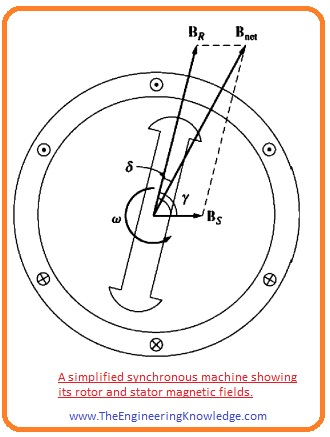Hello, friends, I hope all of you are enjoying your life. In today’s tutorial, I am going to explain Induced Torque in AC Machine. Every alternating current (AC) machine during steady-state working has 2 fields in the machine first one is the static part field and the other is the field in the rotor. These two fields when interacting with each other torque induces in the machine. Torque produces because at some point these two fields cross each other and at some point, add up due to this they produce net force to rotates the rotor.

I have also written an article on the torque induced in the simple loop, it will help you to understand the complicated windings of the machine which has large no of turns. In today’s post, we will have a look at how torque induced in the machine, its equation, working and some other related factor. So, let’s started with How to calculate the induced torque in the AC Machine.

#### Induced Torque AC Machine

• To find the induced torque in the AC machine, the structure of the AC machine is shown in a given diagram, which has flux sinusoidal spread on the stator and its rotor has a single wire coil mounted on the rotor.• The spreading of the stator flux in the machine is given as.
```

```

Bs(α)= Bs(sinα)

• In this equation, the (Bs) is the maximum flux. The value of the term Bs(α) will be positive (+) when the direction of the rotor flux is to the stator.
• To find the value of torque induced in the rotor of a given machine structure, we will examine the torque (tind) and force (F) on both conductors of rotor’s loop one by one.
• The force on the ist conductor of the rotor’s loop is given as.

F = i(l x B)

=ilB(sinα) in the specified direction

• The torque on the first conductor will be.

Tind = (r x F)

=rilBs(sinα) in the clockwise direction.

• The force on the conductor 2 will be given as

F = i(l x B)

=ilB(sinα )

• it will be in the counter-clockwise direction.
• So, the net torque induced on the rotor winding loop will be.
• Tind =2rilBs(sinα) it will be in the anticlockwise direction.• The net torque induced equation in the rotor can be modified by analyzing the given diagram. And made two outcomes for it.
1. The current passing through the winding of the rotor generates the field around the rotor. The direction of this field can be found by the Right Handle Rule, (which says if we grasp a conductor then the curl fingers show the direction of the current and the thumb will be in the direction of the field). And the magnitude of the field intensity (HR) will be directly proportionate to the current passing through the rotor.

(HR) =Ci

• In this equation the (C) is constant.
• Among the maximum value of the stator flux (Bs) and the maximum value of the rotor field intensity (HR) is represented by and given as.

= 1800

sinγ =sin (1800 – α)= sinα

• If we combine these 2 facts, then the induced torque on the rotor can be given as.
• Tind = KHRBSSinα in the anticlockwise direction.
• In the equation, the K is the constant its value relies on the assembly of the machine.
• Direction and the magnitude of the induced torque can be written as.

Tind =KHR x BS

• As we know that BR = HR
• The torque can be written as.

Tind= kBR x BS — (A)

• In this equation the k=K/u. you can see that the (k) will not have the same value.
• As the magnetic permeability (u) changes with the variation in the magnetic saturation in the machine.
• The equation (A) is the same as the equation which we calculate for the single loop in the magnetic field.
• It can apply to any AC machine for the torque measurements. But the only value of (k) will vary from one machine to another apparatus.
• This expression we can use for the quality study of the induced torque in the different AC machines, so the value of the k has not importance for us.
• The entire field in the machine is the summation of the stator and rotor field.

Bnet =BR + Bs —-(B)

• This factor we can use to find the corresponding equation for the torque produced in the machine.
• As we find an equation for the torque. Tind= kBR x BS
• From equation B we can write the expression for field stator.

Bs= Bnet – BR

• Putting this value on the torque equation. We have

Tind=kBR x (Bnet -BR)

= k (BR x Bnet)- k (BR x BR)

• As we already know that cross product of any module with itself is 0 so this equation will become.

Tind =kBR x Bnet

Tind =kBRBnetSinδ

• δ is the value of the angle among the BR and B
• The given below equations can use to comprehensive learning of the torque in alternating current machines.

Tind =kBR x Bs

Tind =kBRBnetSinδ• The field of this machine is moving in the anti-clockwise direction.
• If we apply a right-hand rule to given below equations, then we can find the torque produced in the clockwise direction or reverse the movement direction of the rotor.

Tind =kBR x Bs

Tind =kBR x Bnet

• Consequently, this machine should be work as a generator.

So friends that is the detailed post on the induced voltage in AC machine I try my level best to simplify this tutorial for you. If you have any questions about it ask in comments. See you in the next tutorial.